# 31 Year NEET Previous Year Questions: Nuclei - 1

## 30 Questions MCQ Test Physics 31 Years NEET Chapterwise Solved Papers | 31 Year NEET Previous Year Questions: Nuclei - 1

Description
Attempt 31 Year NEET Previous Year Questions: Nuclei - 1 | 30 questions in 60 minutes | Mock test for NEET preparation | Free important questions MCQ to study Physics 31 Years NEET Chapterwise Solved Papers for NEET Exam | Download free PDF with solutions
QUESTION: 1

### In the reaction ,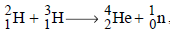if the binding energies of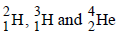are respectively, a, b and c (in MeV), then the energy (in MeV) released in this reaction is 

Solution: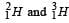requires a and b amount of energies for their nucleons to be separated.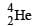releases c amount of energy in its formation i.e., in assembling the nucleons as nucleus.
Hence, Energy released =c – (a + b) = c – a – b

QUESTION: 2

### In any fission process, the ratio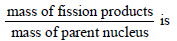Solution:

Binding energy per nucleon for fission products is higher relative to Binding energy per nucleon for parent nucleus, i.e., more masses are lost and are obtained as kinetic energy of fission products. So, the given ratio < 1.

QUESTION: 3

### Fission of nuclei is possible because thebinding energy per nucleon in them 

Solution:

Binding energy per nucleons decreases with increase in mass numbers.
The decrease of binding energy per nucleon for nuclei with a high mass number is due to increased Coulombic repulsion between protons inside the nucleus.

QUESTION: 4

The binding energy of deuteron is 2.2 MeV and that of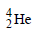is 28 MeV. If two deuterons are fused to form onethen the energy released is          

Solution: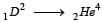Energy released = 28 – 2 × 2.2 = 23.6 MeV (Binding energy is energy released on formation of Nucleus)

QUESTION: 5

In a radioactive material the activity at time t1 is R1 and at a later time t2, it is R2. If the decay constant of the material is λ, then 

Solution:

Let at time t1 & t2, number of particles be N1 & N2. So,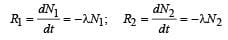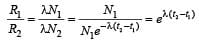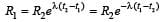QUESTION: 6

Two radioactive substances A and B have decay constants 5λ and λ respectively. At t = 0 they have the same number of nuclei. The ratio of number of nuclei of A to those of B will be (1/e)2 after a time interval 

Solution:

λ A = 5λ and λB = λ
At t = 0,  (N0)A = (N0)B

Given,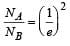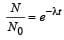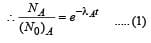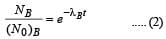From (1) and (2),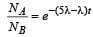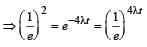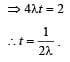QUESTION: 7

In a radioactive decay process, the negativelycharged emitted β -particles are 

Solution:

In beta min us decay (β–), a neutron is transformed into a proton, and an electron is emitted from the nucleus along with antineutrino.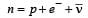QUESTION: 8

A nucleus AZX has mass represented by M (A, Z). If Mp and Mn denote the mass of proton and neutron respectively and B.E. the binding energy in MeV, then 

Solution:

In the case of formation of a nucleus, the evolution of energy equal to the binding energy of the nucleus takes place due to the disappearance of a fraction of the total mass. If the quantity of mass disappearing is ΔM, then the binding energy is

BE = ΔMc2
From the above discussion, it is clear that the mass of the nucleus must be less than the sum of the masses of the constituent neutrons and protons. We can then write.

ΔM= [ZMp + (A-Z)Mn -M(A,Z)

Where M (A, Z)  is the mass of the atom of mass number A and atomic number Z. Hence, the binding energy of the nucleus is

BE = [ZMp + (A-Z)Mn -M(A,Z)]c2

Where N = A -Z = number of neutrons.

QUESTION: 9

If the nucleus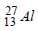has nuclear radius of about 3.6 fm, then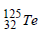would have its radius approximately as 

Solution:

It has been known that a nucleus of mass number A has radius R = R0A1/3,
where R0 = 1.2 × 10–15m and A = mass number

In case of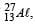let nuclear radius be R1

and for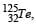nuclear radius be R2

ForFor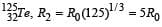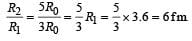QUESTION: 10

Two radioactive materials X1 and X2 have decay constants 5λ and λ respectively. If initially they have the same number of nuclei, then the ratio of the number of nuclei of X1 to that of X2 will be 1/e after a time

Solution:

Let the required time be t. Then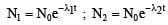Where N1 = number of nuclei of X1 after time t
N2 = number of nuclei of X2 after time t
N0 = initial number of nuclei of X1 and X2 each.

Now,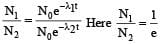λ1 = 5λ;    λ2 = λ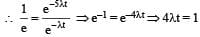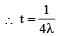QUESTION: 11

If M (A; Z), Mp and Mn denote the masses of the nucleus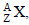proton and neutron respectively in units of u ( 1u = 931.5 MeV/c2) and BE represents its bonding energy in MeV, then 

Solution:

Mass defect = ZMp + (A –Z)Mn–M(A,Z)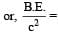ZMp + (A–Z) Mn–M(A,Z)

∴ M (A, Z) = ZMp + (A–Z)Mn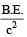QUESTION: 12

Two nuclei have their mass numbers in the ratioof 1 : 3. The ratio of their nuclear densities wouldbe 

Solution:

Requird ratio of nuclear densities =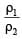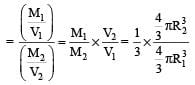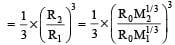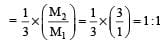QUESTION: 13

In the nuclear decay given below: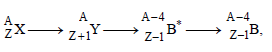the particles emitted in the sequence are

Solution: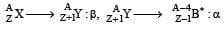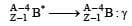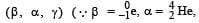mass number and charge number of a nucleus remains unchanged during γ decay)

QUESTION: 14

The mass of a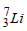nucleus is 0.042 u less than the sum of the masses of all its nucleons. The binding energy per nucleon ofnucleus is nearly

Solution: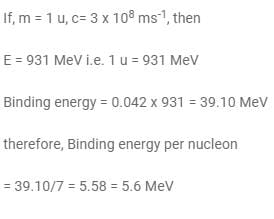QUESTION: 15

The activity of a radioactive sample is measured as N0 counts per minute at t = 0 and N0/e counts per minute at t = 5 minutes. The time (in minutes) at which the activity reduces to half its value is   

Solution: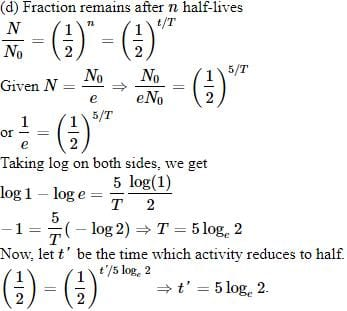QUESTION: 16

The binding energy per nucleon in deuteriumand helium nuclei are 1.1 MeV and 7.0 MeV,respectively. When two deuterium nuclei fuseto form a helium nucleus the energy released inthe fusion is :

Solution:

Binding energy of two 1H2 nuclei = 2(1.1 × 2) = 4.4 MeV
Binding energy of one 2He4 nucelus = 4 × 7.0 =28 MeV
∴ Energy released = 28 – 4.4 = 23.6 MeV

QUESTION: 17

The decay constant of a radio isotope is λ. If A1 and A2 are its activities at times t1 and t2 respectively, the number of nuclei which have decayed during the time (t1 – t2) :

Solution:

Activity is given by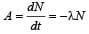Activity at time t1 is A1 =- λN1
and activity at time t2 is A2 = – l N2 As t1 > t2, therefore, number of atoms remained after time t1 is less than that remained after time t2. That is, N1 < N2.
∴ number of nuclei decayed in (t1 – t2)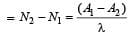QUESTION: 18

The half life of a radioactive isotope 'X' is 50 years. It decays to another element 'Y' which isstable. The two elements 'X' and 'Y' were foundto be in the ratio of 1 : 15 in a sample of agiven rock. The age of the rock was estimatedto be 

Solution: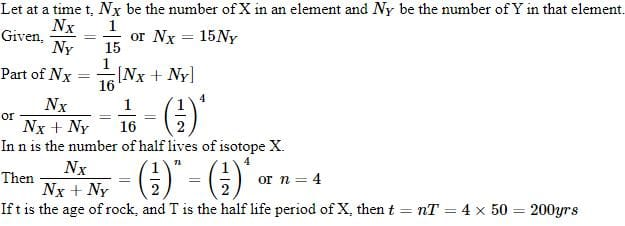QUESTION: 19

The power obtained in a reactor using U235 disintegration is 1000 kW. The mass decay of U235 per hour is 

Solution:

E = mc2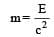So, mass decay per second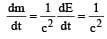(Power in watt)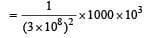and mass decay per hour =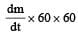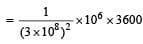= 4 × 10–8 kg

= 40 microgram

QUESTION: 20

A radioactive nucleus of mass M emits a photonof frequency μ and the nucleus recoils. The recoilenergy will be 

Solution:

Momentum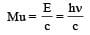Recoil energy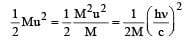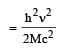QUESTION: 21

A nucleus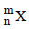emits one α-particle and two β-particles. The resulting nucleus is 

Solution:

When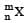emits one -particle then its atomic mass decreases by 4 units and atomic number by 2. Therefore, the new nucleus becomes mn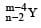. But as it emits two β particles, its atomic number increases by 2. Thus the resulting nucleus is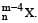QUESTION: 22

Fusion reaction takes place at high temperaturebecause    

Solution:

When the coulomb repulsion between the nuclei is overcome then nuclear fusion reaction takes place. This is possible when temperature is too high.

QUESTION: 23

Two radioactive nuclei P and Q, in a given sample decay into a stable nucleolus R. At time t = 0, number of P species are 4 N0 and that of Q are N0. Half-life of P (for conversion to R) is 1 minute where as that of Q is 2 minutes. Initially there are no nuclei of R present in the sample. When number of nuclei of P and Q are equal, the number of nuclei of R present in the sample would be [2011M]

Solution:

Initially  P → 4N0
Q → N0
Half life TP = 1 min.
TQ = 2 min.
Let after time t number of nuclei of P and Q are equal, that is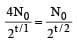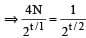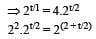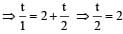⇒ t = 4 min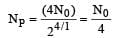at t = 4 min.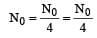or population of R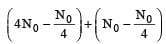QUESTION: 24

If the nuclear radius of 27Al is 3.6 Fermi, the approximate nuclear radius of 64Cu in Fermi is :      

Solution:

The radius of the nuclears is directly proportional to cube root of  atomic number i.e. R ∝ A1/3 ⇒ R = R0 A1/3, where R0 is a constant of proportionality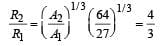where R1 = the radius of 27Al, and A1 = Atomic  mass number of Al R2 = the radius of 64Cu and A2 = Atomic mass number of C4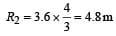QUESTION: 25

A mixture consists of two radioactive materials A1 and A2 with half lives of 20 s and 10 srespectively. Initially the mixture has 40 g of Aand 160 g of A2. The amount of the two in themixture will become equal after : 

Solution:

Let, the amount of the two in the mixture will become equal after t years.
The amount of A1, which remains after t years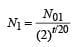The amount of A2, which remains, after t years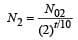According to the problem N1 = N2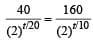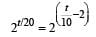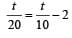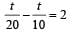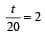t = 40 s

QUESTION: 26

The half life of a radioactive nucleus is 50 days.  The time interval (t2 – t1) between the time twhen 2/3 of its has decayed and the time t1 when 1/3  of it had decayed is :      

Solution: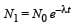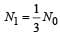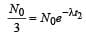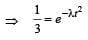...(i)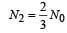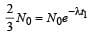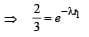...(ii)

Dividing equation (i) by equation (ii)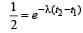λ (t2 - t1) = ln2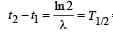= 50 days

QUESTION: 27

A certain mass of Hydrogen is changed to Heliumby the process of fusion. The mass defect infusion reaction is 0.02866 a.m.u. The energyliberated per a.m.u. is(Given : 1 a.m.u = 931 MeV)  [NEET 2013]

Solution:

Mass defect Δm = 0.02866 a.m.u.
Energy = 0.02866 × 931 = 26.7 MeV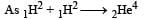Energy liberated per a.m.u = 13.35/2 MeV
= 6.675 MeV

QUESTION: 28

The half life of a radioactive isotope ‘X’ is20 years. It decays to another element ‘Y’ whichis stable. The two elements ‘X’ and ‘Y’ were foundto be in the ratio of 1 : 7 in a sample of a thegiven rock. The age of the rock is estimated tobe [NEET 2013]

Solution:

The value of x is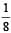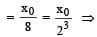t = 3T = 3 × 20 = 60
years Hence the estimated age of the rock is 60 years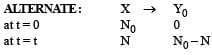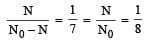t = 3T = 3 × 20 = 60 years

QUESTION: 29

α-particles, β-particles and γ-rays are all havingsame energy. Their penetrating power in a givenmedium in increasing order will be   [NEET Kar. 2013]

Solution:

Increasing order of penetrating power : α < β < γ.
For same energy, lighter particle has higher penentrating power.

QUESTION: 30

How does the binding energy per nucleon varywith the increase in the number of nucleons  ?   [NEET Kar. 2013]

Solution: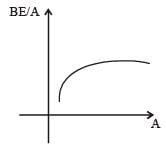From the graph of BE/A versus mass number A  it is clear that, BE/A first increases and then decreases with increase in mass number.Use Code STAYHOME200 and get INR 200 additional OFF Use Coupon Code